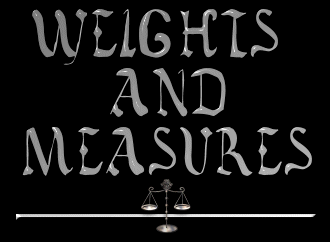Measurements

Ah-il: a unit of measure, the distance from the elbow to the tip of the middle finger, c. 18"; analogous to the Earth cubit; 10 ah-il equal one ah-ral

Ah-ral: a unit of measure equaling 10 ah-il, or approx. 180"

Foot: a unit of measurement equal to 10 horts, or 12 1/2 Earth inches.

Hort: a measure of distance equal to 1- 1/4 inches

Huda: a unit of measure equaling 5 tefa

Pasang: measure of distance equaling .7 miles

Stone: a unit of measurement equal to 4 Earth pounds

Talu: a unit a measure equaling 2 gallons

Tef: a unit of measure, consisting of a handful of whatever produce (such as dates) is being weighed; 6 tefs equal one tefa

Tefa: a unit of measure equaling 6 tefs, or handfuls; 5 tefas equal 1 huda

Weight: a unit of measurement equal to 10 Stone, or 40 Earth lbs.Council ~ Newsletter ~ Members ~ Application
Inactive Members ~ Quotes ~ Guidelines
City Goods ~ Gorean Prices ~ Gorean Money
Weights & Measures ~ Messageboard ~ Yesterday's Merchants